Courses

# Test: Past 28 Years Questions: Ray Optics and Optical Instruments- 1

## 30 Questions MCQ Test Physics Class 12 | Test: Past 28 Years Questions: Ray Optics and Optical Instruments- 1

Description
This mock test of Test: Past 28 Years Questions: Ray Optics and Optical Instruments- 1 for NEET helps you for every NEET entrance exam. This contains 30 Multiple Choice Questions for NEET Test: Past 28 Years Questions: Ray Optics and Optical Instruments- 1 (mcq) to study with solutions a complete question bank. The solved questions answers in this Test: Past 28 Years Questions: Ray Optics and Optical Instruments- 1 quiz give you a good mix of easy questions and tough questions. NEET students definitely take this Test: Past 28 Years Questions: Ray Optics and Optical Instruments- 1 exercise for a better result in the exam. You can find other Test: Past 28 Years Questions: Ray Optics and Optical Instruments- 1 extra questions, long questions & short questions for NEET on EduRev as well by searching above.
QUESTION: 1

### A light ray falls on a rectangular glass slab as shown. The index of refraction of the glass, if total internal reflection is to occur at the vertical face, is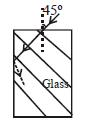Solution: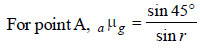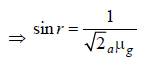For point B, sin (90° – r) = gμa where,
(90° – r) is critical angle.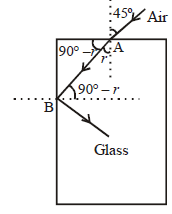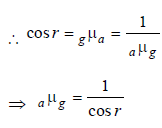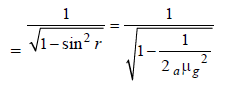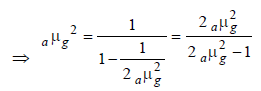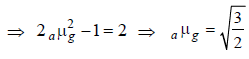QUESTION: 2

### A body is located on a wall. Its image of equal size is to be obtained on a parallel wall with the help of a convex lens. The lens is placed at a distance 'd' ahead of second wall, then the required focal length will be 

Solution:

Using the lens formula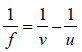Given v = d, for equal size image |v | =|u|  d
By sign convention u = –d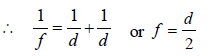QUESTION: 3

### An equiconvex lens is cut into two halves along (i) XOX' and (ii) YOY' as shown in the figure. Let f, f ', f '' be the focal lengths of the complete lens, of each half in case (i), and of each half in case (ii), respectively.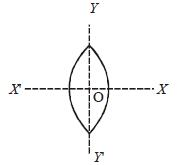Choose the correct statement from the following

Solution: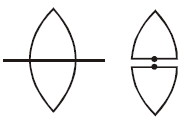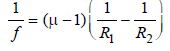In this case, R1 and R2 are unchanged
So, f will remain unchanged for both pieces
of the lens
∴ f = f '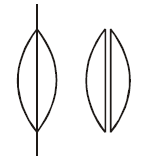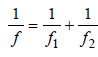This is combination of two lenses of equal
focal lengths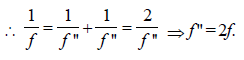QUESTION: 4

A convex lens is dipped in a liquid whoserefractive index is equal to the refractive index ofthe lens. Then its focal length will 

Solution: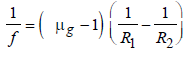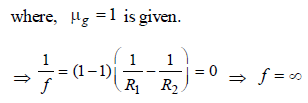QUESTION: 5

The refractive index of the material of a prism is√2 and its refracting angle is 30º. One of therefracting surfaces of the prism is made a mirrorinwards. A beam of monochromatic light entersthe prism from the mirrored surface, then its angleof incidence of the prism is 

Solution:

∠r = 30° (using law of triangle)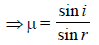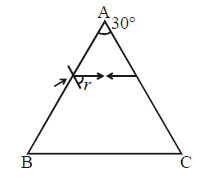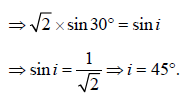QUESTION: 6

A beam of light composed of red and green raysis incident obliquely at a point on the face ofrectangular glass slab. When coming out onthe opposite parallel face, the red and green raysemerge from         

Solution:

Since refractive index for both the light are
different, so they emerge out moving in two
different parallel directions.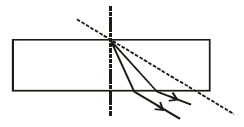QUESTION: 7

A telescope has an objective lens of 10 cmdiameter and is situated at a distance of onekilometer from two objects. The minimumdistance between these two objects, which canbe resolved by the telescope, when the meanwavelength of light is 5000 Å, is of the order of             

Solution: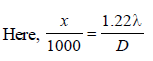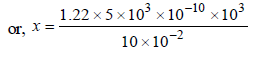or, x = 1.22 × 5 × 10–3 m = 6.1 mm
∴ x is of the order of 5 mm.

QUESTION: 8

The angular resolution of a 10 cm diametertelescope at a wavelength of 5000 Å is of theorder of     

Solution:

Two-point sources are regarded as just resolved when the principal diffraction maximum of one image coincides with the first minimum of the other.
If one considers diffraction through a circular aperture, this translates into the expression
θ=1.22 λ/d​=1.22×(5000×10−8/10​)=1.22×5×10−6=6×10−6rad
Hence, the closest option is 10−6rad

QUESTION: 9

A convex lens and a concave lens, each havingsame focal length of 25 cm, are put in contact toform a combination of lenses. The power indiopters of the combination is  

Solution:

From the formula,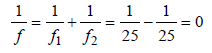Power of combination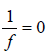QUESTION: 10

A microscope is focussed on a mark on a pieceof paper and then a slab of glass of thickness 3cm and refractive index 1.5 is placed over themark. How should the microscope be moved toget the mark in focus again ? 

Solution:

In the later case microscope will be focussed for O'. So, it is required to be lifted by distance OO'.

OO' = real depth of O – apparent depth of O.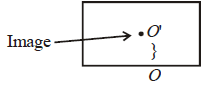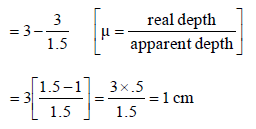QUESTION: 11

The frequency of a light wave in a material is2 × 1014 Hz and wavelength is 5000 Å. Therefractive index of material will be 

Solution:

By using v = nλ
Here, n = 2 x1014Hz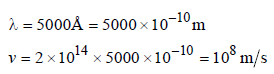Refractive index of the material,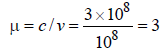QUESTION: 12

A small coin is resting on the bottom of a beaker filled with liquid. A ray of light from the coin travels upto the surface of the liquid and moves along its surface. How fast is the light travelling in the liquid?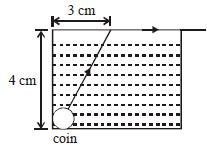Solution: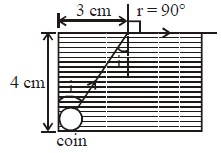Hypotenuse comes out to be 5 cm.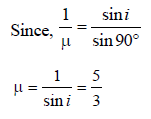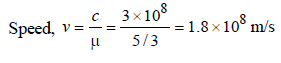QUESTION: 13

Two thin lenses of focal lengths f1 and f2 are in contact and coaxial. The power of the combination is: 

Solution:

The focal length of the combination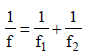∴ Power of the combinations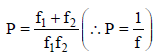QUESTION: 14

A boy is trying to start a fire by focusing sunlighton a piece of paper using an equiconvex lens offocal length 10 cm. The diameter of the Sun is 1.39 ×109 m and its mean distance from the earthis 1.5 × 1011 m. What is the diameter of the Sun’simage on the paper? 

Solution:

We have,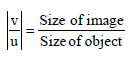or, Size of image =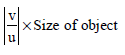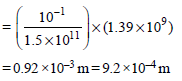∴ Diameter of the sun’s image = 9.2 × 10–4 m.

QUESTION: 15

A ray of light travelling in a transparent mediumof refractive index μ , falls on a surface separatingthe medium from air at an angle of incidence of450 . For which of the following value of μ theray can undergo total internal reflection? 

Solution:

For total internal reflection,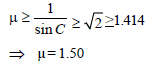QUESTION: 16

Which of the following is not due to total internalreflection?    

Solution:

Difference between apparent and real depth
of a pond is due to the refraction of light,
not due to the total internal reflection. Other
three phenomena are due to the total
internal reflection.

QUESTION: 17

A biconvex lens has a radius of curvature ofmagnitude 20 cm. Which one of the followingoptions best describe the image formed of anobject of height 2 cm placed 30 cm from the lens?   

Solution:

R = 20 cm
h0 = 2
u = –30 cm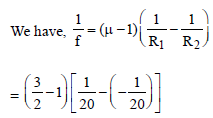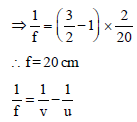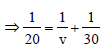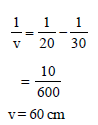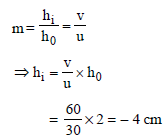QUESTION: 18

A thin prism of angle 15º made of glass ofrefractive index μ1 = 1.5 is combined with anotherprism of glass of refractive index μ2 = 1.75. Thecombination of the prism produces dispersionwithout deviation. The angle of the second prismshould be [2011M]

Solution:

Deviation = zero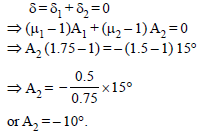or A2 = – 10°.
Negative sign shows that the second prism
is inverted with respect to the first.

QUESTION: 19

A converging beam of rays is incident on adiverging lens. Having passed through the lensthe rays intersect at a point 15 cm from the lenson the opposite side. If the lens is removedthe point where the rays meet will move 5 cmcloser to the lens. The focal length of the lensis [2011M]

Solution: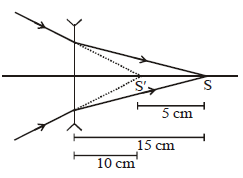By lens formula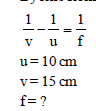Putting the values, we get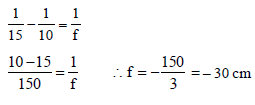QUESTION: 20

When a biconvex lens of glass having refractiveindex 1.47 is dipped in a liquid, it acts as a planesheet of glass. This implies that the liquid musthave refractive index. 

Solution: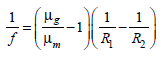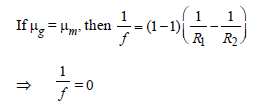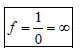QUESTION: 21

A ray of light is incident at an angle of incidence, i, on one face of prism of angle A (assumed to be small) and emerges normally from the opposite face. If the refractive index of the prism is μ, the angle of incidence i, is nearly equal to: 

Solution:

For normally emerge e = 0
Therefore r2 = 0 and r1 = A
Snell’s Law for Incident ray’s
1 sin i = μ sin r1 = μsin A
For small angle
i = μA

QUESTION: 22

f1’ is placed ata distance of 'd’ from a convex lens of focal length ‘f2’. A beam of light coming from infinity andfalling on this convex lens-concave mirrorcombination returns to infinity. The distance ‘d’ must equal :    

Solution: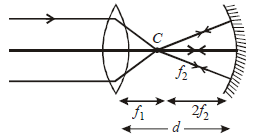d= f1 + 2f2

QUESTION: 23

The magnifying power of a telescope is 9. Whenit is adjusted for parallel rays the distancebetween the objective and eyepiece is 20 cm.The focal length of lenses are :    

Solution: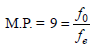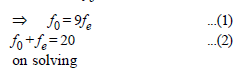f0 = 18 cm = focal length of the objective
fe = 2 cm = focal length of the eyepiece

QUESTION: 24

For the angle of minimum deviation of a prism tobe equal to its refracting angle, the prism mustbe made of a material whose refractive index :            [2012M]

Solution: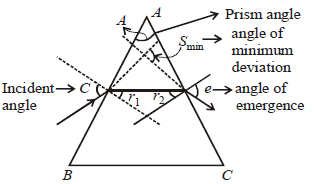The angle of minimum deviation is given as
δmin = i + e–A
for minimum deviation
δmin = A then
2A = i + e
in case of δmin i = e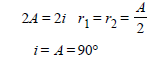from smell’s law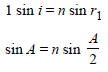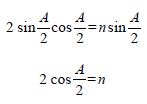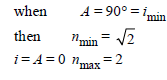QUESTION: 25

A rod of length 10 cm lies along the principalaxis of a concave mirror of focal length 10 cm insuch a way that its end closer to the pole is20 cm away from the mirror. The length of theimage is : [2012M]

Solution: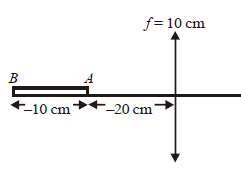The focal length of the mirror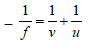For A end of the rod the image distance
When u1 = – 20 cm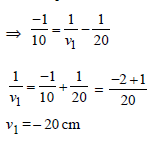For when u2 = – 30 cm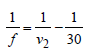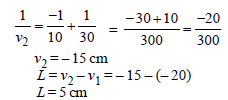QUESTION: 26

The condition under which a microwave ovenheats up a food item containing water moleculesmost efficiently is [NEET 2013]

Solution:

Required condition : Frequency of
microwaves = Resonant frequency of
water molecules.

QUESTION: 27

A plano convex lens fits exactly into a plano concave lens. Their plane surfaces are parallel to each other. If lenses are made of different materials of refractive indices μ1 and μ2 and R is the radius of curvature of the curved surface of the lenses, then the focal length of the combination is [NEET 2013]

Solution: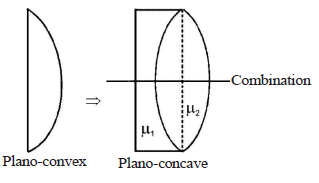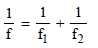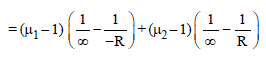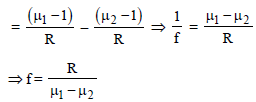Hence, focal length of the combination is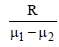QUESTION: 28

For a normal eye, the cornea of eye provides aconverging power of 40D and the leastconverging power of the eye lens behind thecornea is 20D. Using this information, thedistance between the retina and the eye lens ofthe eye can be estimated to be [NEET 2013]

Solution:

Power of Cornea, P1​=40D
and power of the eye lens, P2​=20D
Peff​=P1​+P2
⇒Peff​=40D+20D=60D
So, f= ​100/ Peff ​=100​/60=1.67cm

QUESTION: 29

Two plane mirrors are inclined at 70°. A rayincident on one mirror at angle θ after reflectionfalls on second mirror and is reflected from thereparallel to first mirror. The value of θ is[NEET Kar. 2013]

Solution: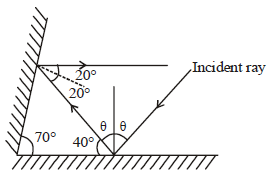From fig. 40° + θ = 90° ∴ θ = 90° – 40° = 50°

QUESTION: 30

The reddish appearance of the sun at sunriseand sunset is due to [NEET Kar. 2013]

Solution:

It is due to scattering of light. Scattering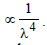Hence the light reaches us is rich in
red